# High School Math : Finding the Center and Radius

## Example Questions

### Example Question #3 : Circle Functions

Find the center and radius of the circle defined by the equation: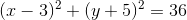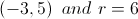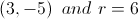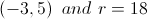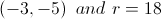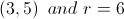Explanation:

The equation of a circle is: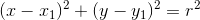whereis the radius and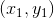is the center.

In this problem, the equation is already in the format required to determine center and radius. To find the-coordinate of the center, we must find the value ofthat makes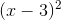equal to 0, which is 3. We do the same to find the y-coordinate of the center and find that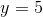. To find the radius we take the square root of the constant on the right side of the equation which is 6.

### Example Question #4 : Circle Functions

Find the center and radius of the circle defined by the equation: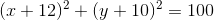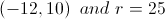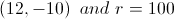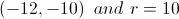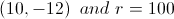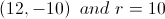The equation of a circle is:whereis the radius andis the center.
In this problem, the equation is already in the format required to determine center and radius. To find the-coordinate of the center, we must find the value ofthat makes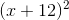equal to, which is. We do the same to find the y-coordinate of the center and find that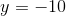. To find the radius we take the square root of the constant on the right side of the equation which is 10.